# SAT II Math II : Solving Trigonometric Functions

## Example Questions

### Example Question #1 : Solving Trigonometric Functions

Give the period of the graph of the equation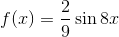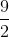The correct answer is not among the other choices.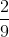The correct answer is not among the other choices.

Explanation:

The period of the graph of a sine function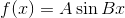is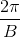, or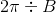.

Since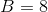,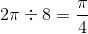.

This answer is not among the given choices.

### Example Question #2 : Solving Trigonometric Functions

If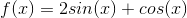, what must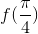be?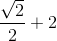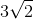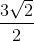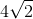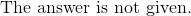Explanation:

Evaluate each trig function at the specified angle.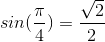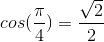Replace the terms into the function.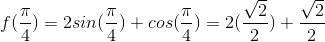Combine like-terms.

The answer is: